0

# Python_sklearn_CountVectorizer使用详解

## 简介

CountVectorizer官方文档。

## 数据预处理

``````import jieba
import re
from sklearn.feature_extraction.text import CountVectorizer
#原始数据
text =['很少在公众场合手机外放','大部分人都还是很认真去学习的','他们会用行动来','无论你现在有多颓废，振作起来','只需要一点点地改变','你的外在和内在都能焕然一新']#提取中文
text =[' '.join(re.findall('[\u4e00-\u9fa5]+',tt,re.S))for tt in text]#分词
text =[' '.join(jieba.lcut(tt))for tt in text]
text
``````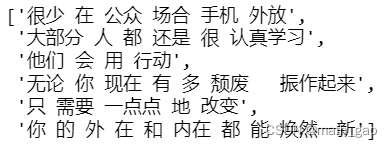## 训练模型

``````#构建模型
vectorizer = CountVectorizer()#训练模型
X = vectorizer.fit_transform(text)
``````

## 所有词汇：model.get_feature_names()

``````#所有文档汇集后生成的词汇
feature_names = vectorizer.get_feature_names()print(feature_names)
``````
1. 不分词生成的词汇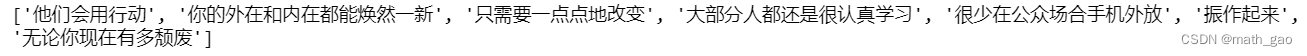2. 分词后生成的词汇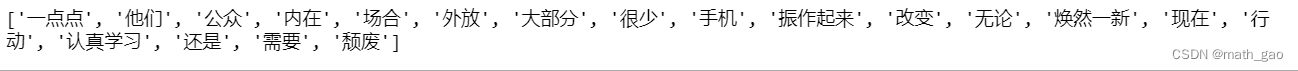## 计数矩阵：X.toarray()

``````#每个文档相对词汇量出现次数形成的矩阵
matrix = X.toarray()print(matrix)
``````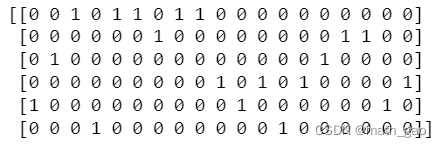``````#计数矩阵转化为DataFrame
df = pd.DataFrame(matrix, columns=feature_names)
df
``````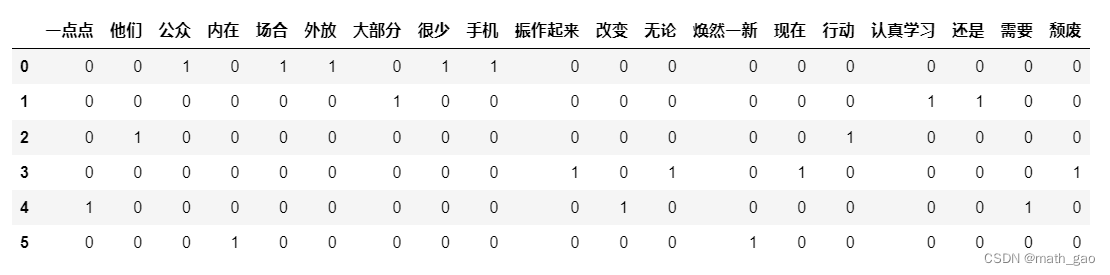## 词汇索引：model.vocabulary_

``````print(vectorizer.vocabulary_)
``````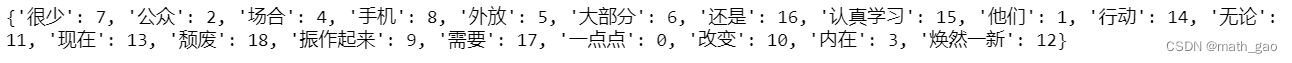### “Python_sklearn_CountVectorizer使用详解”的评论:

##### 关于作者##### overfit同步小助手